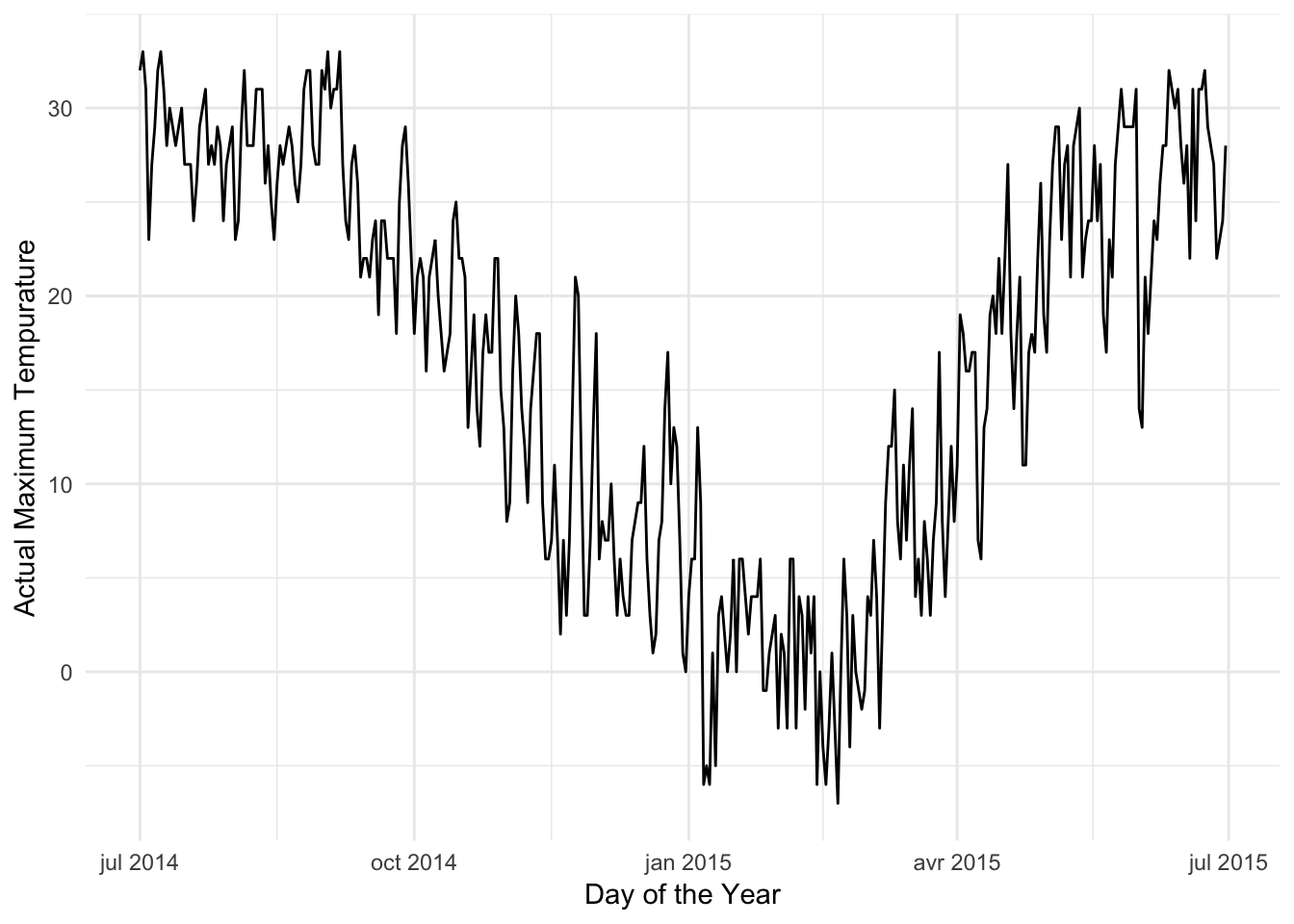## Weather in NYC

The dataset for this practice exam consists of a single year of observed weather data from New York City. Read in the dataset with this command:

``weather <- read_csv("https://statsmaths.github.io/stat_data/weather-nyc.csv")``
``````## Parsed with column specification:
## cols(
##   date = col_date(format = ""),
##   actual_mean_temp = col_double(),
##   actual_min_temp = col_double(),
##   actual_max_temp = col_double(),
##   average_min_temp = col_double(),
##   average_max_temp = col_double(),
##   record_min_temp = col_double(),
##   record_max_temp = col_double(),
##   record_min_temp_year = col_double(),
##   record_max_temp_year = col_double(),
##   actual_precipitation = col_double(),
##   average_precipitation = col_double(),
##   record_precipitation = col_double()
## )``````

Here is a data dictionary for the variables:

• date: the date of the weather record, formatted YYYY-MM-DD
• actual_mean_temp: the measured average temperature for that day (celsius)
• actual_min_temp: the measured minimum temperature for that day (celsius)
• actual_max_temp: the measured maximum temperature for that day (celsius)
• average_min_temp: the average minimum temperature on that day since 1880 (celsius)
• average_max_temp: the average maximum temperature on that day since 1880 (celsius)
• record_min_temp: the lowest ever temperature on that day since 1880 (celsius)
• record_max_temp: the highest ever temperature on that day since 1880 (celsius)
• record_min_temp_year: the year that the lowest ever temperature occurred
• record_max_temp_year: the year that the highest ever temperature occurred
• actual_precipitation: the measured amount of rain or snow for that day (cm)
• average_precipitation: the average amount of rain or snow on that day since 1880 (cm)
• record_precipitation: the highest amount of rain or snow on that day since 1880 (cm)

If other questions about what these variables mean arise, please let me know.

## 1. A straightforward line plot

In the first plot, create a line plot (i.e., use `geom_line`) with date on the x-axis and the actual maximum tempurature on the y-axis. Make sure that you label the two axes. Otherwise, you can leave everything else equal to the defaults.

``````ggplot(weather, aes(date, actual_max_temp)) +
geom_line() +
xlab("Day of the Year") +
ylab("Actual Maximum Tempurature")``````## 2. Two lines on one plot with colors

In the second plot, layer two line geometries that show the actual minimum tempurature and the actual maximum tempurature. Date is still on the x-axis. Further, color the minimum tempurature with the color “#5be5e5” (it’s a pretty shade of blue) and the maximum tempurature with the color “#ff6666” (a pretty shade of red). Don’t forget to include labels for the axes; you do not need to add manual labels for the two curves (it should be relatively obvious what they are showing).

``````ggplot(weather, aes(date, actual_max_temp)) +
geom_line(color = "#ff6666") +
geom_line(aes(y = actual_min_temp), color = "#5be5e5") +
xlab("Day of the Year") +
ylab("Actual Extreme Tempurature")``````